# 7（PHP数组简介）

2021/7/20 13:45:45 浏览：

# 二、数组的定义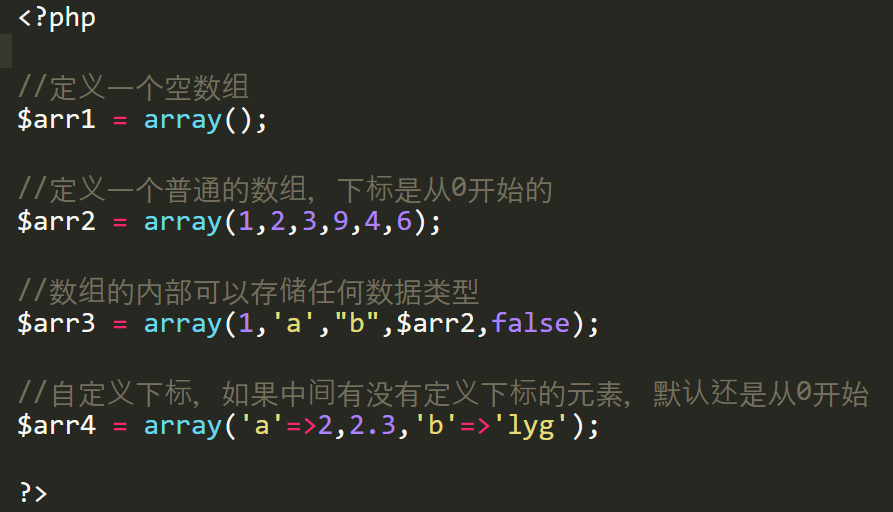# 三、数组的赋值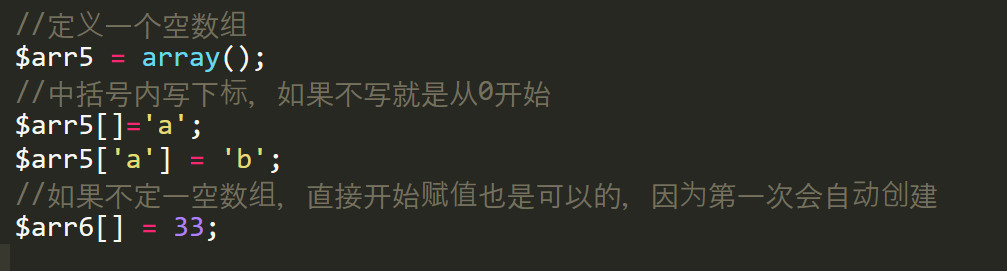# 四、数组的取值

1.可以通过数组的变量名加下标来进行数组的取值：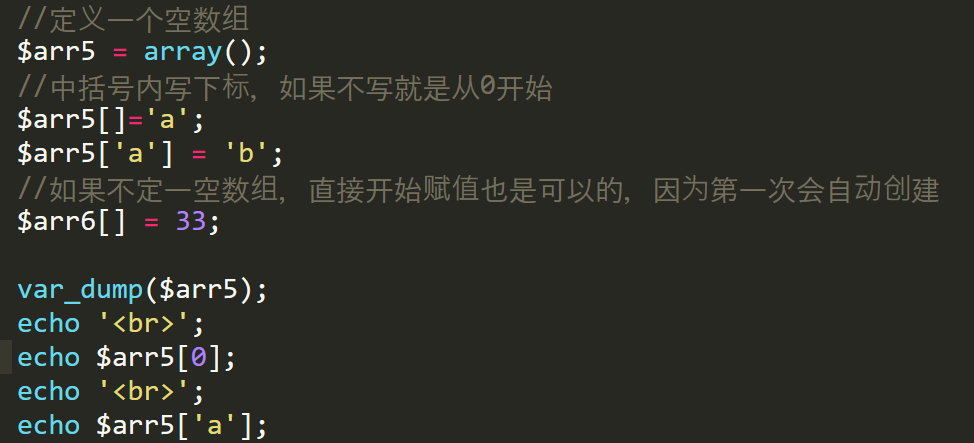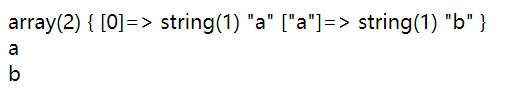2.数组遍历取值。数组的遍历指的是依次将数组的下标和数据取出：

``````\$arr = array(3,’bb’,14,2.1,’a’=>5);

foreach (\$arr  as  \$key => \$value){

//遍历语句；

}``````

\$arr          是数组的变量

as              可以看作是将数组分割为下标和数据

\$key          下标

\$value      数据值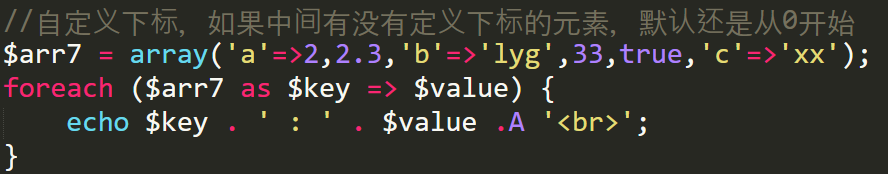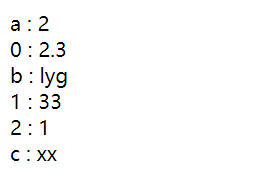# 五、数组的分类

1.按键值关系分:

①索引数组：

数组的下标为从0开始的连续整数的下标，--跟js的数组是一样的

比如：

`` \$arr1 = array(2,3,5,76,8);``

②关联数组：

数组的下标是一个写可以表明数组数据含义的一些字符串；

`` \$emp= array(‘Id’=>1,’Name’=>’小明’,’age’=>22,’salary’=>1000.4);``

2.按复杂程度分：

①一维数组

数组内包含的每一个数据都是一个非数组的值

比如：

`` \$arr2 = array(2,3,5,76,8);``

②二维数组：

数组内包含一个一维数组

比如：

``````\$arr3 = array(

array(1,2,3),

array(4,5,6),

array(7,8,9),

10,
11,
33
);``````

③三维数组或多维数组：

一个数组内嵌套三层或三层以上的数组

# 六、数组常用的部分系统函数

array_pop() : 删除数组的最后一项

array_push() : 将新的一个单元放到数组的最后位置

sort() : 对数组的值按照正序排列

asort() : 对数组的值按照正序排列，并保持键值关系

in_array() : 判断某个数据是否存在于某个数组内

count() : 计数，获得数组的长度

range() : 设定2个数据的界限，并返回这个范围中的所有数据值多对应的数组

array_merge() : 合并数组

``````<?php
\$arr1 = array(1,2,3,9,4,6);
\$arr2 = array(1,'a',"b",\$arr1,false);

//array_pop() : 删除数组的最后一项
var_dump(\$arr1);//array(6) { => int(1) => int(2) => int(3) => int(9) => int(4) => int(6) }
array_pop(\$arr1);
var_dump(\$arr1);//array(5) { => int(1) => int(2) => int(3) => int(9) => int(4) }

//array_push() : 将新的一个单元放到数组的最后位置
array_push(\$arr1,'xinzeng');
var_dump(\$arr1);//array(6) { => int(1) => int(2) => int(3) => int(9) => int(4) => string(7) "xinzeng" }

//sort() : 对数组的值按照正序排列
sort(\$arr1);
var_dump(\$arr1);//array(6) { => string(7) "xinzeng" => int(1) => int(2) => int(3) => int(4) => int(9) }

//asort() : 对数组的值按照正序排列，并保持键值关系
var_dump(\$arr2);//array(5) { => int(1) => string(1) "a" => string(1) "b" => array(6) { => int(1) => int(2) => int(3) => int(9) => int(4) => int(6) } => bool(false) }
asort(\$arr2);
var_dump(\$arr2);//array(5) { => bool(false) => string(1) "a" => string(1) "b" => int(1) => array(6) { => int(1) => int(2) => int(3) => int(9) => int(4) => int(6) } }

echo '<br>'.'--------------------------';
//in_array() : 判断某个数据是否存在于某个数组内
echo in_array(1,\$arr2);//1
echo in_array('ff',\$arr2);//null

//count() : 计数，获得数组的长度
echo count(\$arr2);//5

//range() : 设定2个数据的界限，返回这个范围中的所有数据值对应的数组,例如
//设置了1,5，就会返回一个值为1到5的数组
var_dump(range(1,5));//array(5) { => int(1) => int(2) => int(3) => int(4) => int(5) }

//array_merge() : 合并数组
\$arr01 = array(1,2);
\$arr02 = array(1,3);
var_dump(array_merge(\$arr01,\$arr02));//array(4) { => int(1) => int(2) => int(1) => int(3) }
?>``````